## teaching and educationJOURNAL OFAPPLIEDCRYSTALLOGRAPHY
ISSN: 1600-5767
Volume 48| Part 3| June 2015| Pages 901-905

## Why crystal structure analysis works: a one-dimensional crystallography teaching tool

aDepartment of Chemistry, University of Toledo, Toledo, OH 43606, USA
*Correspondence e-mail: a.pinkerton@utoledo.edu

(Received 23 February 2015; accepted 9 April 2015; online 22 May 2015)

A teaching tool is proposed to help beginner students of crystallography understand how crystallographic calculations work. Examples of the most important methods taught in X-ray crystallography courses have been adapted to a one-dimensional hypothetical structure. All calculations can be carried out in class with a scientific calculator or by using a simple spreadsheet.

### 1. Introduction

One of the challenges in teaching crystallography is to provide a digestible explanation of what goes on inside the very powerful black boxes that we all currently use for structure solution and refinement. The student is typically faced with indigestible mathematical formulae involving triple summations concerning multitudes of atoms and reflections in direct or reciprocal space. Stout & Jensen (1968)showed how the principles of this daunting mathematical landscape could be clarified by examining the calculation of structure factors and Fourier series for a hypothetical one-dimensional one-atom problem. We have taken this approach and extended it to the Patterson function, direct methods and least-squares refinement. By simplifying the three-dimensional expressions to analyze a one-dimensional one-atom problem, it is possible to carry out all relevant calculations with a pocket calculator or simple spreadsheet in class. In common with Stout & Jensen, the chosen one-dimensional structure has a unit-cell length of 10 Å, has an inversion center and contains two carbon atoms at x = ±0.1833, as shown below. The hypothetical experiment was carried out with Cu Kα radiation, λ = 1.5418 Å.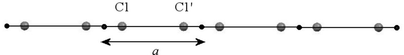As this is proposed as a teaching aid, minimal information is provided to introduce each section, but the transformation of three-dimensional (3D) to one-dimensional (1D) equations is given, followed by numerical or partial numerical examples. For class use, it is simple to remove calculated numbers from the tables to allow hand calculations as exercises or as homework. Details of the underlying theory may be found in a number of standard books or from web-based materials (see http://iycr2014.org/learn/educational-materials).

### 2. Bragg's law

The following table of experimental' observations may be generated for the hypothetical structure, along with the corresponding reciprocal lattice below.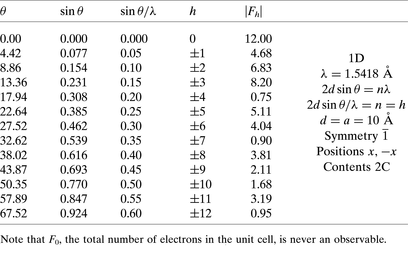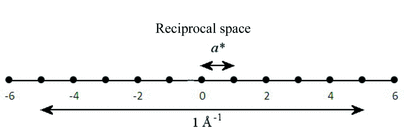### 3. Unit-cell refinement

Given the observed Bragg angles for the series of indexed reflections shown above, we can obtain the best values for the unit-cell dimensions using a linear least-squares approach. The following treatment is numerically equivalent to minimizing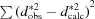.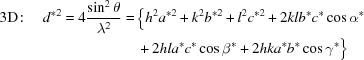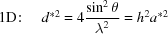For n observations, we can write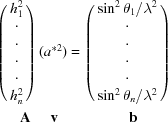We may thus solve for v in the following way: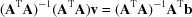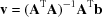Using the values of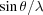and h given above we obtain the results tabulated below: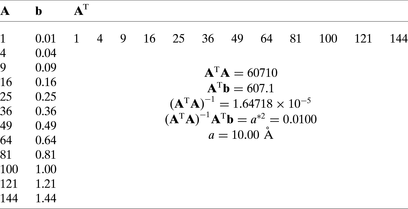### 4. Patterson function

Taking the square of the structure factor amplitudes given above, we may calculate a Patterson map. A partial map is given below. Given the equivalent positions, x, −x, the Patterson vectors will be at 2x, −2x. From the observed maximum at 0.36, we obtain x = 0.18 for one carbon atom.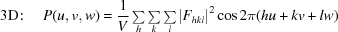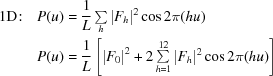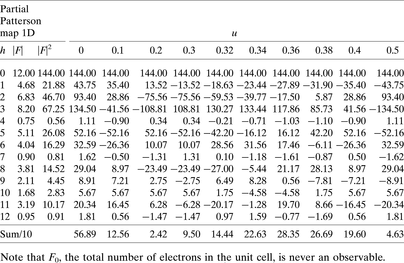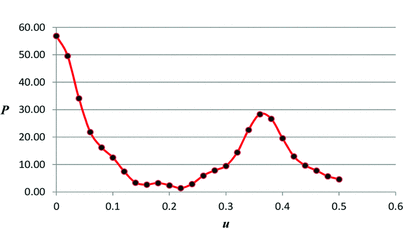### 5. Structure factor calculation

If the structure is known, then we can calculate structure factors from the atomic positions and the scattering power of the atoms. The following example uses the result of the Patterson function [x(C1) = 0.18] to calculate the corresponding structure factors and compare them with their observed values. The comparison of the two sets of values provides the traditional crystallographic R factor.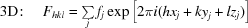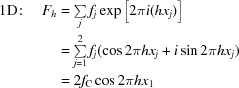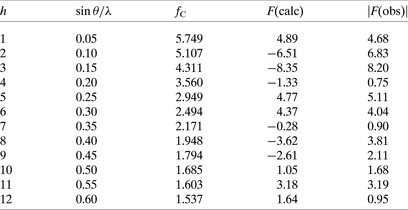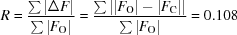### 6. Fourier summation

Using appropriate phases computed as above coupled with the observed structure factor amplitudes, a Fourier map will have maxima at the atomic positions. The details of a partial Fourier summation assuming Friedel's law are shown in the table below, followed by a plot of the function. The maximum is found at x = 0.18.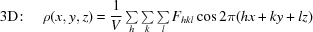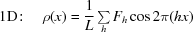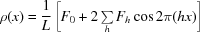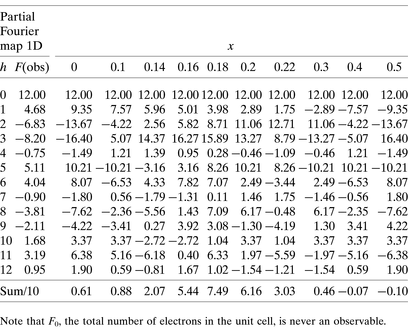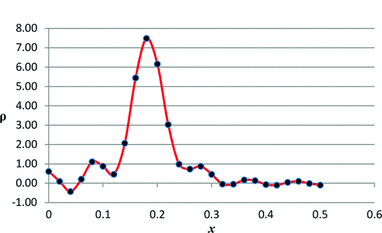### 7. Direct methods

Direct methods for phase determination use probabilities based on normalized structure factors (E), which are calculated from F for stationary point atoms. The following sections define the appropriate E values, show how the origin is defined, employ the probabilities obtained from Σ1 and Σ2 relationships to obtain probable phases for a few reflections, use symbolic addition to extend these phases, and then use this subset of phased E values to compute a Fourier map.

#### 7.1. E values

The following expressions remove the dependence of structure factors onand normalize with respect to the total number of electrons in the structure.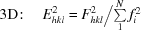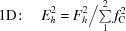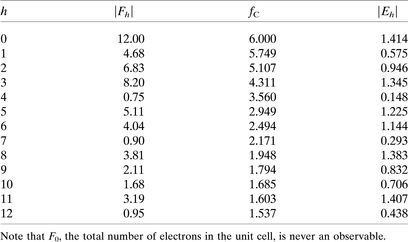#### 7.2. Origin definition

As shown in the following table, the phases of reflections with all even indices are invariant with respect to the choice of origin, whereas all other parities may change phase in a predictable manner. Thus a suitable choice of a few phases may be used to define the origin of the structure.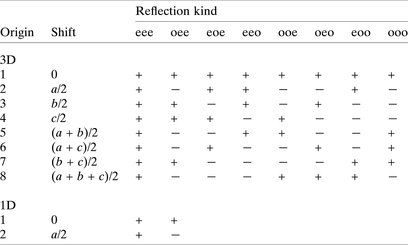#### 7.3. Probabilities from Σ1 and Σ2 relationships

In the following, H and K represent different values of h forming triples, whereas in the three-dimensional case the triples would contain h, k, l. The values of P+ indicate the probability that the product of the phases is positive. For Σ1 (involving only pairs of reflections) the most probable result is highlighted in bold. For Σ2, only a selection of triples has been calculated. Again the most probable results have been highlighted in bold, plus two more of more modest probability in bold italics.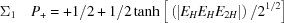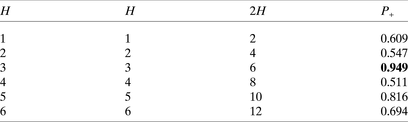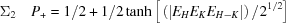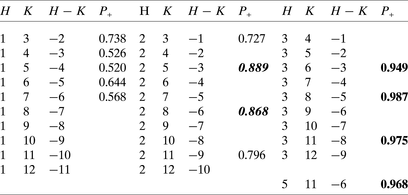#### 7.4. Structure solution

Define origin – choose h odd with high E.

h = 5, E = 1.223, phase +.

Insert known phases for the high-probability triples such that the product is positive, and add symbols for unknowns: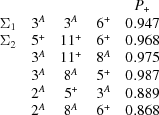Thus A = −, or else all would be + (the uranium' disaster).

Calculate a Fourier map using only the phased E values –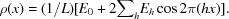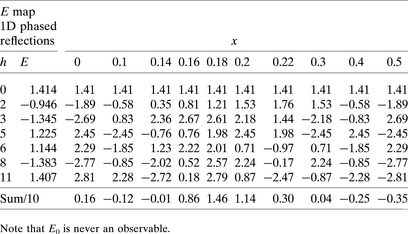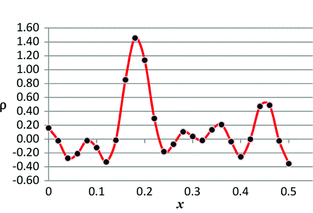Thus the maximum in the density is again at x = 0.18.

### 8. Structure refinement

When atomic positions have been determined by any of the methods described above, it is common practice to refine this structure model using nonlinear least-squares methods. Shifts in atomic coordinates are thus calculated to iteratively obtain the best fit between the model structure and the experimental data. The following equations are mathematically equivalent to minimizing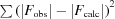. All Fs in the following expressions represent amplitudes.

For the general case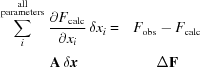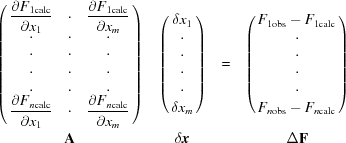For one parameter in one dimension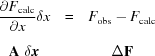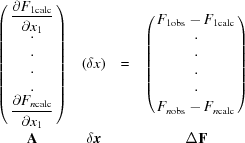We may solve for δx as follows: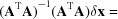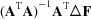, thus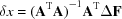.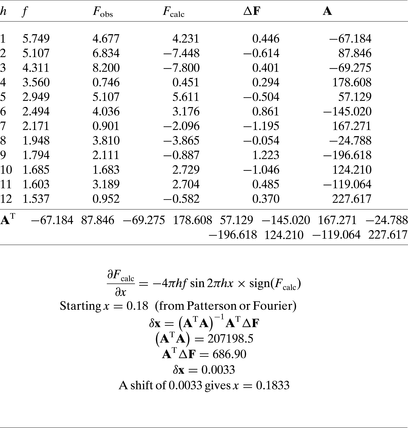### 9. Conclusion

By simple extrapolation of the one-dimensional example proposed by Stout & Jensen (1968), we have provided a teaching tool that covers the basics of structure determination and refinement in a way that should be digestible to a beginner student of X-ray crystallography.

### ReferencesJOURNAL OFAPPLIEDCRYSTALLOGRAPHY